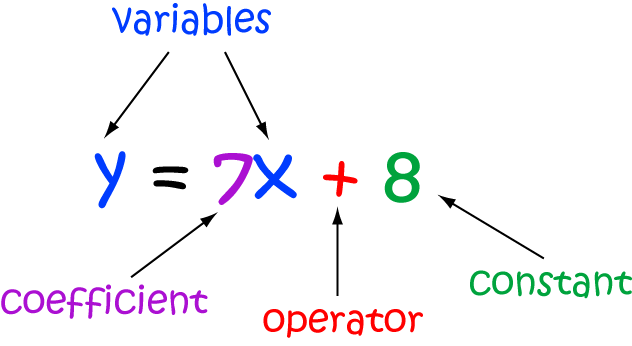# Definition of PronumeralA pronumeral is another word for a "variable"

For example "$x$" and "$y$" are pronumerals in the equation. They can stand for any numbers that satisfy the equation.

### Description

The aim of this dictionary is to provide definitions to common mathematical terms. Students learn a new math skill every week at school, sometimes just before they start a new skill, if they want to look at what a specific term means, this is where this dictionary will become handy and a go-to guide for a student.

### Audience

Year 1 to Year 12 students

### Learning Objectives

Learn common math terms starting with letter P

Author: Subject Coach
You must be logged in as Student to ask a Question.